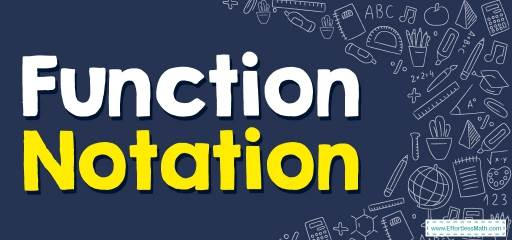# How to Solve Function Notation? (+FREE Worksheet!)

Learn what the definition of function is and how to write a function using function notation and how to evaluate a function.## Step by step guide to solve Function Notation

• Functions are mathematical operations that assign unique outputs to given inputs.
• Function notation is the way a function is written. It is meant to be a precise way of giving information about the function without a rather lengthy written explanation.
• The most popular function notation is $$f(x)$$ which is read “$$f$$ of $$x$$”. Any letter can be used to name a function. for example: $$g(x)$$, $$h(x)$$, etc.
• To evaluate a function, plug in the input (the given value or expression) for the function’s variable (place holder, $$x$$ ).

A Perfect Practice Workbook for Algebra I

### Function Notation – Example 1:

Evaluate. $$h(n)=n^2-2$$
Find $$h(4)$$.

Solution:

Substitute $$(4)$$ for $$n$$ and solve. $$h(n)=n^2-2→h(4)=(4)^2-2→h(4)=16-2=14→h(4)=14$$

### Function Notation – Example 2:

Evaluate. $$w(x)=4x-1$$
Find $$w(2)$$.

Solution:

Substitute $$(2)$$ for $$x$$ and solve. Then: $$w(x)=4x-1→w(2)=4(2)-1→w(2)=8-1=7→w(2)=7$$

### Function Notation – Example 3:

Evaluate. $$w(x)=3x+1$$
Find $$w(4)$$.

Solution:

Substitute $$x$$ with $$4$$: Then: $$w(x)=3x+1→w(4)=3(4)+1→w(4)=12+1=13→w(4)=13$$

Best Algebra Prep Resource

### Function Notation – Example 4:

Evaluate. $$h(n)=n^2-10$$
Find $$h(-2)$$.

Solution:

Substitute $$n$$ with $$-2$$: $$h(n)=n^2-10→h(-2)=(-2)^2-10→h(-2)=4-10=-6→h(-2)=-6$$

## Exercises for Solving Function Notation

### Evaluate each function.

1. $$\color{blue}{w(x) = 3x + 1, find \ w(4)}$$
2. $$\color{blue}{h(n) = n^2 – 10, find \ h(5)}$$
3. $$\color{blue}{h(x) = x^3 + 8, find \ h(–2)}$$
4. $$\color{blue}{h(n) =\space – 2n^2 – 6n, find \ h(2)}$$
5. $$\color{blue}{g(n) = 3n^2 + 2n, find \ g(2)}$$
6. $$\color{blue}{g(n) = 10n\space – 3, find \ g(6)}$$

1. $$\color{blue}{13}$$
2. $$\color{blue}{15}$$
3. $$\color{blue}{0}$$
4. $$\color{blue}{-20}$$
5. $$\color{blue}{16}$$
6. $$\color{blue}{57}$$

The Absolute Best Books to Ace Algebra

### What people say about "How to Solve Function Notation? (+FREE Worksheet!) - Effortless Math: We Help Students Learn to LOVE Mathematics"?

No one replied yet.

X
45% OFF

Limited time only!

Save Over 45%

SAVE $40 It was$89.99 now it is \$49.99